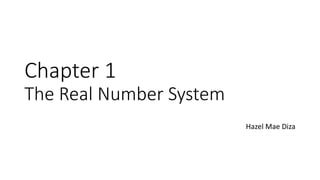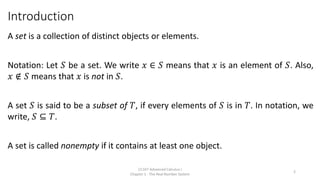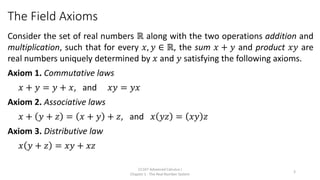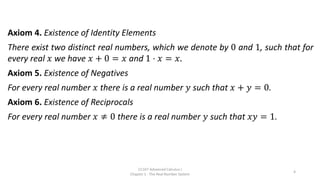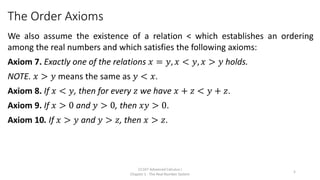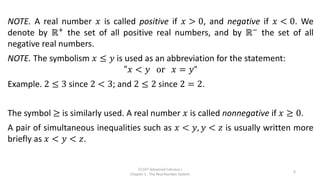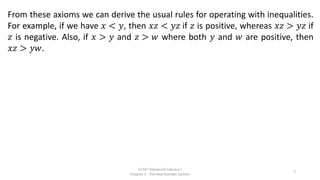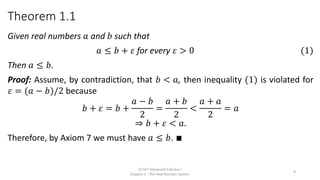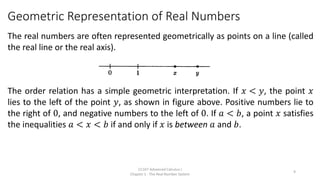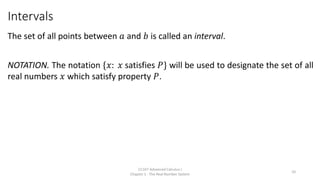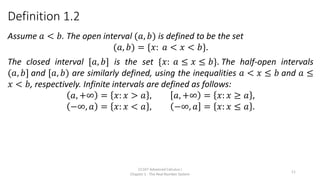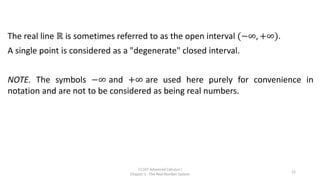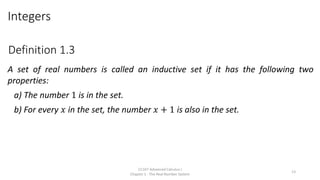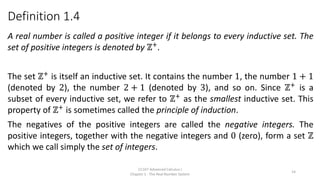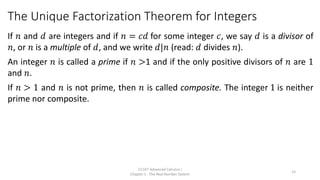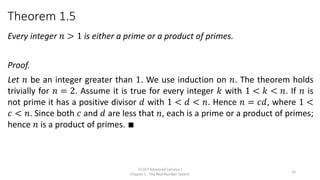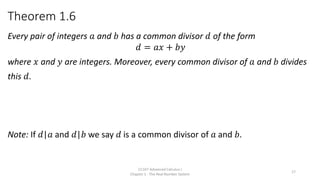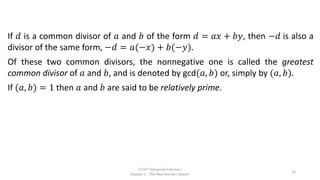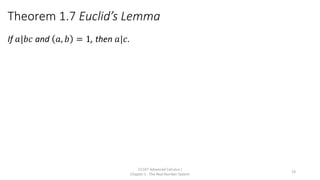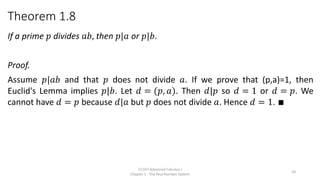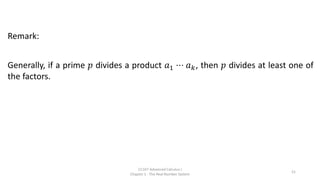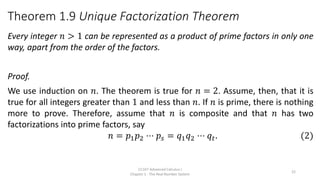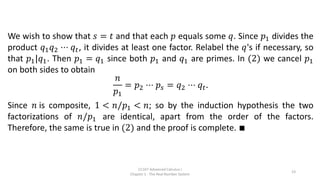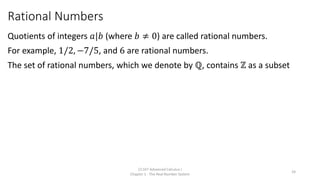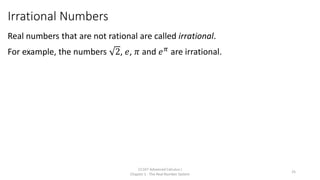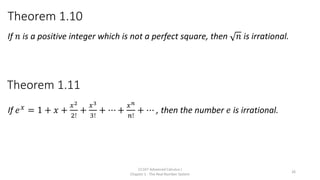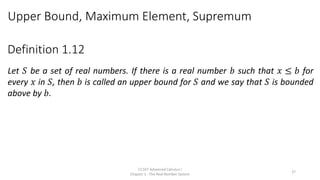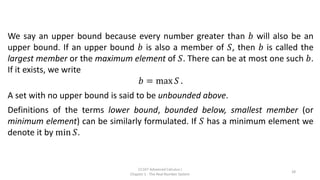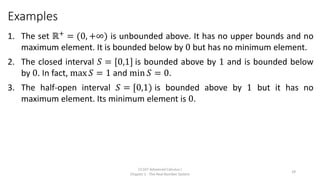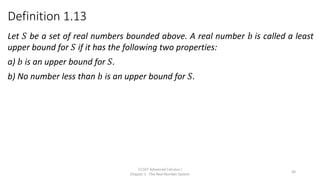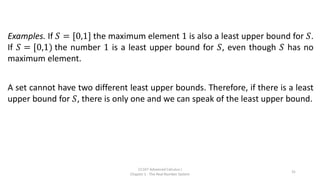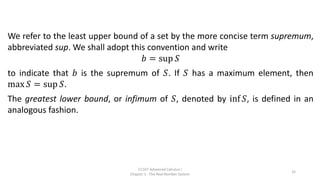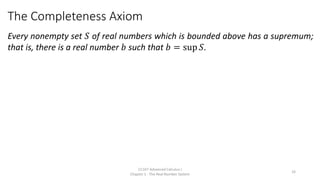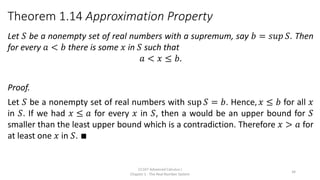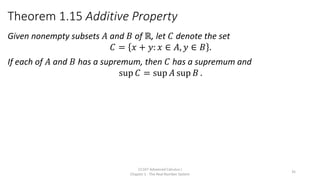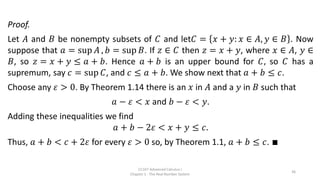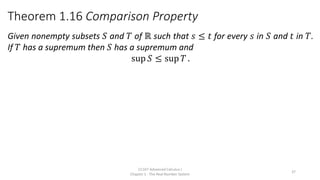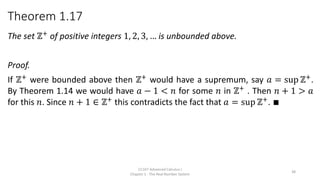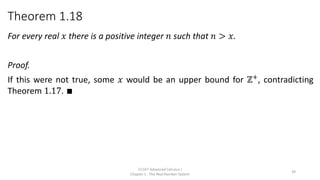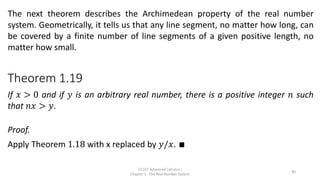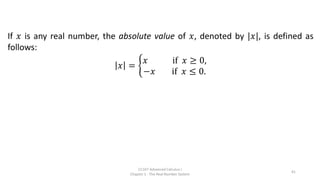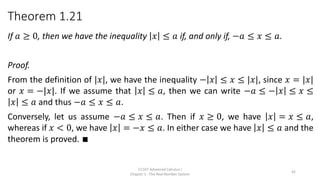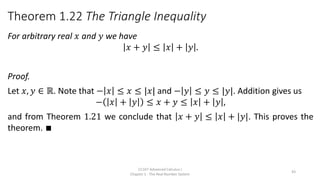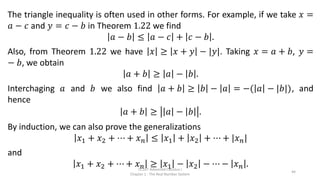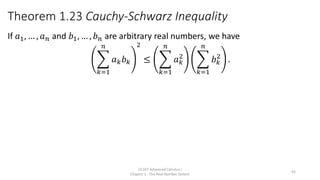1 of 45

### CC107 - Chapter 1 The real Number System.pdf

1. Chapter 1 The Real Number System Hazel Mae Diza
2. Introduction A set is a collection of distinct objects or elements. Notation: Let 𝑆 be a set. We write 𝑥 ∈ 𝑆 means that 𝑥 is an element of 𝑆. Also, 𝑥 ∉ 𝑆 means that 𝑥 is not in 𝑆. A set 𝑆 is said to be a subset of 𝑇, if every elements of 𝑆 is in 𝑇. In notation, we write, 𝑆 ⊆ 𝑇. A set is called nonempty if it contains at least one object. CC107 Advanced Calculus I Chapter 1 - The Real Number System 2
3. The Field Axioms Consider the set of real numbers ℝ along with the two operations addition and multiplication, such that for every 𝑥, 𝑦 ∈ ℝ, the sum 𝑥 + 𝑦 and product 𝑥𝑦 are real numbers uniquely determined by 𝑥 and 𝑦 satisfying the following axioms. Axiom 1. Commutative laws 𝑥 + 𝑦 = 𝑦 + 𝑥, and 𝑥𝑦 = 𝑦𝑥 Axiom 2. Associative laws 𝑥 + 𝑦 + 𝑧 = 𝑥 + 𝑦 + 𝑧, and 𝑥 𝑦𝑧 = 𝑥𝑦 𝑧 Axiom 3. Distributive law 𝑥 𝑦 + 𝑧 = 𝑥𝑦 + 𝑥𝑧 CC107 Advanced Calculus I Chapter 1 - The Real Number System 3
4. Axiom 4. Existence of Identity Elements There exist two distinct real numbers, which we denote by 0 and 1, such that for every real 𝑥 we have 𝑥 + 0 = 𝑥 and 1 ⋅ 𝑥 = 𝑥. Axiom 5. Existence of Negatives For every real number 𝑥 there is a real number 𝑦 such that 𝑥 + 𝑦 = 0. Axiom 6. Existence of Reciprocals For every real number 𝑥 ≠ 0 there is a real number 𝑦 such that 𝑥𝑦 = 1. CC107 Advanced Calculus I Chapter 1 - The Real Number System 4
5. The Order Axioms We also assume the existence of a relation < which establishes an ordering among the real numbers and which satisfies the following axioms: Axiom 7. Exactly one of the relations 𝑥 = 𝑦, 𝑥 < 𝑦, 𝑥 > 𝑦 holds. NOTE. 𝑥 > 𝑦 means the same as 𝑦 < 𝑥. Axiom 8. If 𝑥 < 𝑦, then for every 𝑧 we have 𝑥 + 𝑧 < 𝑦 + 𝑧. Axiom 9. If 𝑥 > 0 and 𝑦 > 0, then 𝑥𝑦 > 0. Axiom 10. If 𝑥 > 𝑦 and 𝑦 > 𝑧, then 𝑥 > 𝑧. CC107 Advanced Calculus I Chapter 1 - The Real Number System 5
6. NOTE. A real number 𝑥 is called positive if 𝑥 > 0, and negative if 𝑥 < 0. We denote by ℝ+ the set of all positive real numbers, and by ℝ− the set of all negative real numbers. NOTE. The symbolism 𝑥 ≤ 𝑦 is used as an abbreviation for the statement: "𝑥 < 𝑦 or 𝑥 = 𝑦“ Example. 2 ≤ 3 since 2 < 3; and 2 ≤ 2 since 2 = 2. The symbol ≥ is similarly used. A real number 𝑥 is called nonnegative if 𝑥 ≥ 0. A pair of simultaneous inequalities such as 𝑥 < 𝑦, 𝑦 < 𝑧 is usually written more briefly as 𝑥 < 𝑦 < 𝑧. CC107 Advanced Calculus I Chapter 1 - The Real Number System 6
7. From these axioms we can derive the usual rules for operating with inequalities. For example, if we have 𝑥 < 𝑦, then 𝑥𝑧 < 𝑦𝑧 if 𝑧 is positive, whereas 𝑥𝑧 > 𝑦𝑧 if 𝑧 is negative. Also, if 𝑥 > 𝑦 and 𝑧 > 𝑤 where both 𝑦 and 𝑤 are positive, then 𝑥𝑧 > 𝑦𝑤. CC107 Advanced Calculus I Chapter 1 - The Real Number System 7
8. Theorem 1.1 Given real numbers 𝑎 and 𝑏 such that 𝑎 ≤ 𝑏 + 𝜀 for every 𝜀 > 0 (1) Then 𝑎 ≤ 𝑏. Proof: Assume, by contradiction, that 𝑏 < 𝑎, then inequality (1) is violated for 𝜀 = (𝑎 − 𝑏)/2 because 𝑏 + 𝜀 = 𝑏 + 𝑎 − 𝑏 2 = 𝑎 + 𝑏 2 < 𝑎 + 𝑎 2 = 𝑎 ⇒ 𝑏 + 𝜀 < 𝑎. Therefore, by Axiom 7 we must have 𝑎 ≤ 𝑏. ∎ CC107 Advanced Calculus I Chapter 1 - The Real Number System 8
9. Geometric Representation of Real Numbers The real numbers are often represented geometrically as points on a line (called the real line or the real axis). The order relation has a simple geometric interpretation. If 𝑥 < 𝑦, the point 𝑥 lies to the left of the point 𝑦, as shown in figure above. Positive numbers lie to the right of 0, and negative numbers to the left of 0. If 𝑎 < 𝑏, a point 𝑥 satisfies the inequalities 𝑎 < 𝑥 < 𝑏 if and only if 𝑥 is between 𝑎 and 𝑏. CC107 Advanced Calculus I Chapter 1 - The Real Number System 9
10. Intervals The set of all points between 𝑎 and 𝑏 is called an interval. NOTATION. The notation {𝑥: 𝑥 satisfies 𝑃} will be used to designate the set of all real numbers 𝑥 which satisfy property 𝑃. CC107 Advanced Calculus I Chapter 1 - The Real Number System 10
11. Definition 1.2 Assume 𝑎 < 𝑏. The open interval (𝑎, 𝑏) is defined to be the set (𝑎, 𝑏) = {𝑥: 𝑎 < 𝑥 < 𝑏}. The closed interval [𝑎, 𝑏] is the set {𝑥: 𝑎 ≤ 𝑥 ≤ 𝑏}. The half-open intervals (𝑎, 𝑏] and [𝑎, 𝑏) are similarly defined, using the inequalities 𝑎 < 𝑥 ≤ 𝑏 and 𝑎 ≤ 𝑥 < 𝑏, respectively. Infinite intervals are defined as follows: 𝑎, +∞ = 𝑥: 𝑥 > 𝑎 , 𝑎, +∞ = 𝑥: 𝑥 ≥ 𝑎 , −∞, 𝑎 = 𝑥: 𝑥 < 𝑎 , −∞, 𝑎 = 𝑥: 𝑥 ≤ 𝑎 . CC107 Advanced Calculus I Chapter 1 - The Real Number System 11
12. The real line ℝ is sometimes referred to as the open interval (−∞, +∞). A single point is considered as a "degenerate" closed interval. NOTE. The symbols −∞ and +∞ are used here purely for convenience in notation and are not to be considered as being real numbers. CC107 Advanced Calculus I Chapter 1 - The Real Number System 12
13. Integers A set of real numbers is called an inductive set if it has the following two properties: a) The number 1 is in the set. b) For every 𝑥 in the set, the number 𝑥 + 1 is also in the set. CC107 Advanced Calculus I Chapter 1 - The Real Number System 13 Definition 1.3
14. Definition 1.4 A real number is called a positive integer if it belongs to every inductive set. The set of positive integers is denoted by ℤ+. The set ℤ+ is itself an inductive set. It contains the number 1, the number 1 + 1 (denoted by 2), the number 2 + 1 (denoted by 3), and so on. Since ℤ+ is a subset of every inductive set, we refer to ℤ+ as the smallest inductive set. This property of ℤ+ is sometimes called the principle of induction. The negatives of the positive integers are called the negative integers. The positive integers, together with the negative integers and 0 (zero), form a set ℤ which we call simply the set of integers. CC107 Advanced Calculus I Chapter 1 - The Real Number System 14
15. The Unique Factorization Theorem for Integers If 𝑛 and 𝑑 are integers and if 𝑛 = 𝑐𝑑 for some integer 𝑐, we say 𝑑 is a divisor of 𝑛, or 𝑛 is a multiple of 𝑑, and we write 𝑑|𝑛 (read: 𝑑 divides 𝑛). An integer 𝑛 is called a prime if 𝑛 >1 and if the only positive divisors of 𝑛 are 1 and 𝑛. If 𝑛 > 1 and 𝑛 is not prime, then 𝑛 is called composite. The integer 1 is neither prime nor composite. CC107 Advanced Calculus I Chapter 1 - The Real Number System 15
16. Theorem 1.5 Every integer 𝑛 > 1 is either a prime or a product of primes. Proof. Let 𝑛 be an integer greater than 1. We use induction on 𝑛. The theorem holds trivially for 𝑛 = 2. Assume it is true for every integer 𝑘 with 1 < 𝑘 < 𝑛. If 𝑛 is not prime it has a positive divisor 𝑑 with 1 < 𝑑 < 𝑛. Hence 𝑛 = 𝑐𝑑, where 1 < 𝑐 < 𝑛. Since both 𝑐 and 𝑑 are less that 𝑛, each is a prime or a product of primes; hence 𝑛 is a product of primes. ∎ CC107 Advanced Calculus I Chapter 1 - The Real Number System 16
17. Theorem 1.6 Every pair of integers 𝑎 and 𝑏 has a common divisor 𝑑 of the form 𝑑 = 𝑎𝑥 + 𝑏𝑦 where 𝑥 and 𝑦 are integers. Moreover, every common divisor of 𝑎 and 𝑏 divides this 𝑑. Note: If 𝑑|𝑎 and 𝑑|𝑏 we say 𝑑 is a common divisor of 𝑎 and 𝑏. CC107 Advanced Calculus I Chapter 1 - The Real Number System 17
18. If 𝑑 is a common divisor of 𝑎 and 𝑏 of the form 𝑑 = 𝑎𝑥 + 𝑏𝑦, then −𝑑 is also a divisor of the same form, −𝑑 = 𝑎(−𝑥) + 𝑏(−𝑦). Of these two common divisors, the nonnegative one is called the greatest common divisor of 𝑎 and 𝑏, and is denoted by gcd(𝑎, 𝑏) or, simply by (𝑎, 𝑏). If (𝑎, 𝑏) = 1 then 𝑎 and 𝑏 are said to be relatively prime. CC107 Advanced Calculus I Chapter 1 - The Real Number System 18
19. Theorem 1.7 Euclid’s Lemma If 𝑎|𝑏𝑐 and 𝑎, 𝑏 = 1, then 𝑎|𝑐. CC107 Advanced Calculus I Chapter 1 - The Real Number System 19
20. Theorem 1.8 If a prime 𝑝 divides 𝑎𝑏, then 𝑝|𝑎 or 𝑝|𝑏. Proof. Assume 𝑝|𝑎𝑏 and that 𝑝 does not divide 𝑎. If we prove that (p,a)=1, then Euclid's Lemma implies 𝑝|𝑏. Let 𝑑 = (𝑝, 𝑎). Then 𝑑|𝑝 so 𝑑 = 1 or 𝑑 = 𝑝. We cannot have 𝑑 = 𝑝 because 𝑑|𝑎 but 𝑝 does not divide 𝑎. Hence 𝑑 = 1. ∎ CC107 Advanced Calculus I Chapter 1 - The Real Number System 20
21. Remark: Generally, if a prime 𝑝 divides a product 𝑎1 ⋅⋅⋅ 𝑎𝑘, then 𝑝 divides at least one of the factors. CC107 Advanced Calculus I Chapter 1 - The Real Number System 21
22. Theorem 1.9 Unique Factorization Theorem Every integer 𝑛 > 1 can be represented as a product of prime factors in only one way, apart from the order of the factors. Proof. We use induction on 𝑛. The theorem is true for 𝑛 = 2. Assume, then, that it is true for all integers greater than 1 and less than 𝑛. If 𝑛 is prime, there is nothing more to prove. Therefore, assume that 𝑛 is composite and that 𝑛 has two factorizations into prime factors, say 𝑛 = 𝑝1𝑝2 ⋅⋅⋅ 𝑝𝑠 = 𝑞1𝑞2 ⋅⋅⋅ 𝑞𝑡. (2) CC107 Advanced Calculus I Chapter 1 - The Real Number System 22
23. We wish to show that 𝑠 = 𝑡 and that each 𝑝 equals some 𝑞. Since 𝑝1 divides the product 𝑞1𝑞2 ⋅⋅⋅ 𝑞𝑡, it divides at least one factor. Relabel the 𝑞's if necessary, so that 𝑝1|𝑞1. Then 𝑝1 = 𝑞1 since both 𝑝1 and 𝑞1 are primes. In (2) we cancel 𝑝1 on both sides to obtain 𝑛 𝑝1 = 𝑝2 ⋅⋅⋅ 𝑝𝑠 = 𝑞2 ⋅⋅⋅ 𝑞𝑡. Since 𝑛 is composite, 1 < 𝑛/𝑝1 < 𝑛; so by the induction hypothesis the two factorizations of 𝑛/𝑝1 are identical, apart from the order of the factors. Therefore, the same is true in (2) and the proof is complete. ∎ CC107 Advanced Calculus I Chapter 1 - The Real Number System 23
24. Rational Numbers Quotients of integers 𝑎|𝑏 (where 𝑏 ≠ 0) are called rational numbers. For example, 1/2, −7/5, and 6 are rational numbers. The set of rational numbers, which we denote by ℚ, contains ℤ as a subset CC107 Advanced Calculus I Chapter 1 - The Real Number System 24
25. Irrational Numbers Real numbers that are not rational are called irrational. For example, the numbers 2, 𝑒, 𝜋 and 𝑒𝜋 are irrational. CC107 Advanced Calculus I Chapter 1 - The Real Number System 25
26. Theorem 1.10 If 𝑛 is a positive integer which is not a perfect square, then 𝑛 is irrational. CC107 Advanced Calculus I Chapter 1 - The Real Number System 26 Theorem 1.11 If 𝑒𝑥 = 1 + 𝑥 + 𝑥2 2! + 𝑥3 3! + ⋯ + 𝑥𝑛 𝑛! + ⋯ , then the number 𝑒 is irrational.
27. Upper Bound, Maximum Element, Supremum Let 𝑆 be a set of real numbers. If there is a real number 𝑏 such that 𝑥 ≤ 𝑏 for every 𝑥 in 𝑆, then 𝑏 is called an upper bound for 𝑆 and we say that 𝑆 is bounded above by 𝑏. CC107 Advanced Calculus I Chapter 1 - The Real Number System 27 Definition 1.12
28. We say an upper bound because every number greater than 𝑏 will also be an upper bound. If an upper bound 𝑏 is also a member of 𝑆, then 𝑏 is called the largest member or the maximum element of 𝑆. There can be at most one such 𝑏. If it exists, we write 𝑏 = max 𝑆 . A set with no upper bound is said to be unbounded above. Definitions of the terms lower bound, bounded below, smallest member (or minimum element) can be similarly formulated. If 𝑆 has a minimum element we denote it by min 𝑆. CC107 Advanced Calculus I Chapter 1 - The Real Number System 28
29. Examples 1. The set ℝ+ = (0, +∞) is unbounded above. It has no upper bounds and no maximum element. It is bounded below by 0 but has no minimum element. 2. The closed interval 𝑆 = [0,1] is bounded above by 1 and is bounded below by 0. In fact, max 𝑆 = 1 and min 𝑆 = 0. 3. The half-open interval 𝑆 = [0,1) is bounded above by 1 but it has no maximum element. Its minimum element is 0. CC107 Advanced Calculus I Chapter 1 - The Real Number System 29
30. Definition 1.13 Let 𝑆 be a set of real numbers bounded above. A real number 𝑏 is called a least upper bound for 𝑆 if it has the following two properties: a) 𝑏 is an upper bound for 𝑆. b) No number less than 𝑏 is an upper bound for 𝑆. CC107 Advanced Calculus I Chapter 1 - The Real Number System 30
31. Examples. If 𝑆 = [0,1] the maximum element 1 is also a least upper bound for 𝑆. If 𝑆 = [0,1) the number 1 is a least upper bound for 𝑆, even though 𝑆 has no maximum element. A set cannot have two different least upper bounds. Therefore, if there is a least upper bound for 𝑆, there is only one and we can speak of the least upper bound. CC107 Advanced Calculus I Chapter 1 - The Real Number System 31
32. We refer to the least upper bound of a set by the more concise term supremum, abbreviated sup. We shall adopt this convention and write 𝑏 = sup 𝑆 to indicate that 𝑏 is the supremum of 𝑆. If 𝑆 has a maximum element, then max 𝑆 = sup 𝑆. The greatest lower bound, or infimum of 𝑆, denoted by inf 𝑆, is defined in an analogous fashion. CC107 Advanced Calculus I Chapter 1 - The Real Number System 32
33. The Completeness Axiom Every nonempty set 𝑆 of real numbers which is bounded above has a supremum; that is, there is a real number 𝑏 such that 𝑏 = sup 𝑆. CC107 Advanced Calculus I Chapter 1 - The Real Number System 33
34. Theorem 1.14 Approximation Property Let 𝑆 be a nonempty set of real numbers with a supremum, say 𝑏 = 𝑠𝑢𝑝 𝑆. Then for every 𝑎 < 𝑏 there is some 𝑥 in 𝑆 such that 𝑎 < 𝑥 ≤ 𝑏. Proof. Let 𝑆 be a nonempty set of real numbers with sup 𝑆 = 𝑏. Hence, 𝑥 ≤ 𝑏 for all 𝑥 in 𝑆. If we had 𝑥 ≤ 𝑎 for every 𝑥 in 𝑆, then a would be an upper bound for 𝑆 smaller than the least upper bound which is a contradiction. Therefore 𝑥 > 𝑎 for at least one 𝑥 in 𝑆. ∎ CC107 Advanced Calculus I Chapter 1 - The Real Number System 34
35. Theorem 1.15 Additive Property Given nonempty subsets 𝐴 and 𝐵 of ℝ, let 𝐶 denote the set 𝐶 = 𝑥 + 𝑦: 𝑥 ∈ 𝐴, 𝑦 ∈ 𝐵 . If each of 𝐴 and 𝐵 has a supremum, then 𝐶 has a supremum and sup 𝐶 = sup 𝐴 sup 𝐵 . CC107 Advanced Calculus I Chapter 1 - The Real Number System 35
36. Proof. Let 𝐴 and 𝐵 be nonempty subsets of 𝐶 and let𝐶 = 𝑥 + 𝑦: 𝑥 ∈ 𝐴, 𝑦 ∈ 𝐵 . Now suppose that 𝑎 = sup 𝐴 , 𝑏 = sup 𝐵. If 𝑧 ∈ 𝐶 then 𝑧 = 𝑥 + 𝑦, where 𝑥 ∈ 𝐴, 𝑦 ∈ 𝐵, so 𝑧 = 𝑥 + 𝑦 ≤ 𝑎 + 𝑏. Hence 𝑎 + 𝑏 is an upper bound for 𝐶, so 𝐶 has a supremum, say 𝑐 = sup 𝐶, and 𝑐 ≤ 𝑎 + 𝑏. We show next that 𝑎 + 𝑏 ≤ 𝑐. Choose any 𝜀 > 0. By Theorem 1.14 there is an 𝑥 in 𝐴 and a 𝑦 in 𝐵 such that 𝑎 − 𝜀 < 𝑥 and 𝑏 − 𝜀 < 𝑦. Adding these inequalities we find 𝑎 + 𝑏 − 2𝜀 < 𝑥 + 𝑦 ≤ 𝑐. Thus, 𝑎 + 𝑏 < 𝑐 + 2𝜀 for every 𝜀 > 0 so, by Theorem 1.1, 𝑎 + 𝑏 ≤ 𝑐. ∎ CC107 Advanced Calculus I Chapter 1 - The Real Number System 36
37. Theorem 1.16 Comparison Property Given nonempty subsets 𝑆 and 𝑇 of ℝ such that 𝑠 ≤ 𝑡 for every 𝑠 in 𝑆 and 𝑡 in 𝑇. If 𝑇 has a supremum then 𝑆 has a supremum and sup 𝑆 ≤ sup 𝑇 . CC107 Advanced Calculus I Chapter 1 - The Real Number System 37
38. Theorem 1.17 The set ℤ+ of positive integers 1, 2, 3, … is unbounded above. Proof. If ℤ+ were bounded above then ℤ+ would have a supremum, say 𝑎 = sup ℤ+. By Theorem 1.14 we would have 𝑎 − 1 < 𝑛 for some 𝑛 in ℤ+ . Then 𝑛 + 1 > 𝑎 for this 𝑛. Since 𝑛 + 1 ∈ ℤ+ this contradicts the fact that 𝑎 = sup ℤ+ . ∎ CC107 Advanced Calculus I Chapter 1 - The Real Number System 38
39. Theorem 1.18 For every real 𝑥 there is a positive integer 𝑛 such that 𝑛 > 𝑥. Proof. If this were not true, some 𝑥 would be an upper bound for ℤ+, contradicting Theorem 1.17. ∎ CC107 Advanced Calculus I Chapter 1 - The Real Number System 39
40. Theorem 1.19 If 𝑥 > 0 and if 𝑦 is an arbitrary real number, there is a positive integer 𝑛 such that 𝑛𝑥 > 𝑦. Proof. Apply Theorem 1.18 with x replaced by 𝑦/𝑥. ∎ CC107 Advanced Calculus I Chapter 1 - The Real Number System 40 The next theorem describes the Archimedean property of the real number system. Geometrically, it tells us that any line segment, no matter how long, can be covered by a finite number of line segments of a given positive length, no matter how small.
41. If 𝑥 is any real number, the absolute value of 𝑥, denoted by |𝑥|, is defined as follows: 𝑥 = ቊ 𝑥 if 𝑥 ≥ 0, −𝑥 if 𝑥 ≤ 0. CC107 Advanced Calculus I Chapter 1 - The Real Number System 41
42. Theorem 1.21 If 𝑎 ≥ 0, then we have the inequality 𝑥 ≤ 𝑎 if, and only if, −𝑎 ≤ 𝑥 ≤ 𝑎. Proof. From the definition of |𝑥|, we have the inequality − 𝑥 ≤ 𝑥 ≤ |𝑥|, since 𝑥 = |𝑥| or 𝑥 = −|𝑥|. If we assume that 𝑥 ≤ 𝑎, then we can write −𝑎 ≤ − 𝑥 ≤ 𝑥 ≤ 𝑥 ≤ 𝑎 and thus −𝑎 ≤ 𝑥 ≤ 𝑎. Conversely, let us assume −𝑎 ≤ 𝑥 ≤ 𝑎. Then if 𝑥 ≥ 0, we have 𝑥 = 𝑥 ≤ 𝑎, whereas if 𝑥 < 0, we have 𝑥 = −𝑥 ≤ 𝑎. In either case we have 𝑥 ≤ 𝑎 and the theorem is proved. ∎ CC107 Advanced Calculus I Chapter 1 - The Real Number System 42
43. Theorem 1.22 The Triangle Inequality For arbitrary real 𝑥 and 𝑦 we have 𝑥 + 𝑦 ≤ 𝑥 + 𝑦 . Proof. Let 𝑥, 𝑦 ∈ ℝ. Note that − 𝑥 ≤ 𝑥 ≤ |𝑥| and − 𝑦 ≤ 𝑦 ≤ |𝑦|. Addition gives us − 𝑥 + 𝑦 ≤ 𝑥 + 𝑦 ≤ 𝑥 + 𝑦 , and from Theorem 1.21 we conclude that 𝑥 + 𝑦 ≤ 𝑥 + |𝑦|. This proves the theorem. ∎ CC107 Advanced Calculus I Chapter 1 - The Real Number System 43
44. The triangle inequality is often used in other forms. For example, if we take 𝑥 = 𝑎 − 𝑐 and 𝑦 = 𝑐 − 𝑏 in Theorem 1.22 we find 𝑎 − 𝑏 ≤ 𝑎 − 𝑐 + 𝑐 − 𝑏 . Also, from Theorem 1.22 we have 𝑥 ≥ 𝑥 + 𝑦 − |𝑦|. Taking 𝑥 = 𝑎 + 𝑏, 𝑦 = − 𝑏, we obtain 𝑎 + 𝑏 ≥ 𝑎 − 𝑏 . Interchaging 𝑎 and 𝑏 we also find 𝑎 + 𝑏 ≥ 𝑏 − 𝑎 = −( 𝑎 − |𝑏|), and hence 𝑎 + 𝑏 ≥ 𝑎 − 𝑏 . By induction, we can also prove the generalizations 𝑥1 + 𝑥2 + ⋯ + 𝑥𝑛 ≤ 𝑥1 + 𝑥2 + ⋯ + |𝑥𝑛| and 𝑥1 + 𝑥2 + ⋯ + 𝑥𝑛 ≥ 𝑥1 − 𝑥2 − ⋯ − 𝑥𝑛 . CC107 Advanced Calculus I Chapter 1 - The Real Number System 44
45. Theorem 1.23 Cauchy-Schwarz Inequality If 𝑎1, … , 𝑎𝑛 and 𝑏1, … , 𝑏𝑛 are arbitrary real numbers, we have ෍ 𝑘=1 𝑛 𝑎𝑘𝑏𝑘 2 ≤ ෍ 𝑘=1 𝑛 𝑎𝑘 2 ෍ 𝑘=1 𝑛 𝑏𝑘 2 . CC107 Advanced Calculus I Chapter 1 - The Real Number System 45